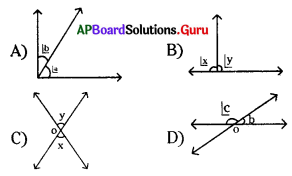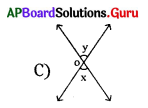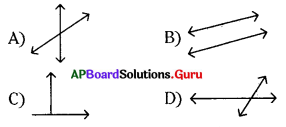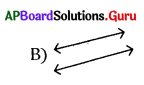Practice the AP 7th Class Maths Bits with Answers Chapter 4 Lines and Angles on a regular basis so that you can attempt exams with utmost confidence.

## AP State Syllabus 7th Class Maths Bits 4th Lesson Lines and Angles with Answers

Question 1.
If p//q and ∠1 – 55° then ∠2 =
A) 55°
B) 35°
C) 145°
D) 110°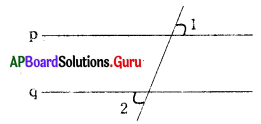A) 55°

Question 2.
The complementary angle for 72° is
A) 108°
B) 18°
C) 28°
D) 118°
B) 18°Question 3.
The supplementary angle for 65° is
A) 35°
B) 45°
C) 25°
D) 115°
D) 115°

Question 4.
In the figure the value of x is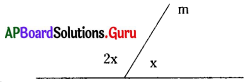A) 60°
B) 120°
C) 90°
D) 180°
A) 60°

Question 5.
In the figure l//m then the value of x is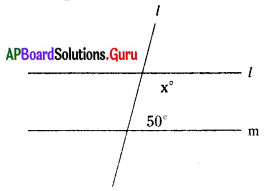A) 50°
B) 40°
C) 130°
D) 160°
C) 130°

Question 6.
In the figure p//q then ∠a =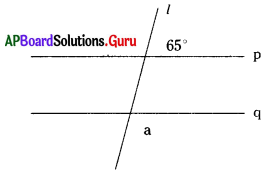A) 65°
B) 25°
C) 115°
D) 35°
C) 115°

Question 7.
The complementary angle of 45° is …………..
A) 90°
B) 45°
C) 135°
D) 55°
B) 45°

Question 8.
The complementary angle of 89° is ……………
A) 1°
B) 90°
C) 91°
D) 9°
A) 1°

Question 9.
If two angles are complement to each other and are equal, then the angles are?
A) (60°, 60°)
B) (89°, 89°)
C) (45°, 45°)
D) (90°, 90°)
C) (45°, 45°)Question 10.
The supplementary angle of 135° is …………….?
A) 35°
B) 65°
C) 55°
D) 45°
D) 45°

Question 11.
If two angles are equal and supplement to each other, then they are ……………
A)(120°, 120°)
B) (60°, 120°)
C) (45°, 135°)
D) (90°, 90°)
D) (90°, 90°)

Question 12.
The supplement of 30° is …………….
A) 140°
B) 160°
C) 150°
D) 60°
C) 150°

Question 13.
Number of transversal lines can be drawn to two distinct lines are ……………?
A) 1
B) 2
C) infinite
D) None
C) infinite

Question 14.
A transversal line intersects two distinct lines in …………… distinct points.
A) 0
B) 1
C) 2
D) infinite
C) 2

Question 15.
From the adjacent figure ⌊x° = …………..?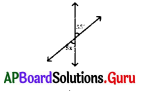A) 10°
B) 11°
C) 55°
D) 5°
B) 11°

Question 16.
From the adjacent figure ⌊y – [⌊x + ⌊z] = …………….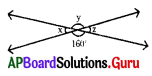A) 120°
B) 140°
C) 20°
D) 180°
A) 120°Question 17.
From the adjacent figure ⌊x = ………………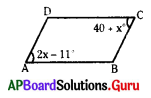A) 61°
B) 51°
C) 41°
D) 50°
B) 51°

Question 18.
From the adjacent figure ⌊p = …………….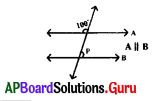A) 100°
B) 70°
C) 80°
D) 60°
C) 80°

Question 19.
From the given figure l || BC, ⌊x + ⌊z = ……………….?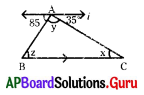A) 35°
B) 85°
C) 60°
D) 120°
D) 120°

Question 20.
From the given figure ⌊Q = ?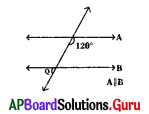A) 120°
B) 60°
C) 70°
D) 30°
B) 60°

Question 21.
In the given figure one pair of adjacent angle is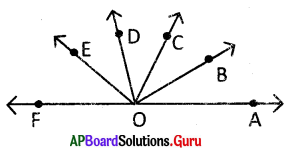A) ∠AOB, ∠COD
B) ∠AOB, ∠AOC
C) ∠FOE, ∠FOD
D) ∠EOD, ∠DOC
D) ∠EOD, ∠DOC

Question 22.
Which of the following pairs of angles are not complementary?
A) (45°, 55°)
B) (35°, 55°)
C) (23°, 67°)
D) (49°, 41°)
A) (45°, 55°)

Question 23.
Which of the following pairs of angles are supplementary angles?
A) (90°, 89°)
B) (105°, 85°)
C) (125°, 55°)
D) (64°, 136°)
C) (125°, 55°)Question 24.
Which of the following is not correct?
A) Each angle in any pair of complementary angles is always acute.
B) Two acute angles can’t form a supplementary angle.
C) Two right angles form a supplementary angle.
D) If the sum of two angles is supplementary then each of them is an obtuse angle.
D) If the sum of two angles is supplementary then each of them is an obtuse angle.

Question 25.
From the given figure. A pair of adjacent angles is?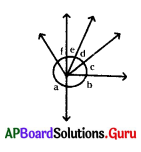A) ⌊a, ⌊c
B) ⌊d, ⌊f
C) ⌊b, ⌊e
D) ⌊f, ⌊a
D) ⌊f,⌊a

Question 26.
Which of the following is an acute angle?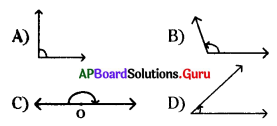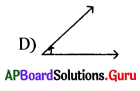Question 27.
Which of the following is not true?
A) Any pair of supplementary angles are adjacent.
B) Any pair of adjacent angles form a supplementary angle.
C) Any pair of complementary angles are adjacent.
D) Any pair of adjacent angles doesn’t form a complementary angle.
B) Any pair of adjacent angles form a supplementary angle.

Question 28.
If two angles are supplementary then they are
A) both acute
B) both obtuse
C) one acute and one obtuse
D) can’t be determined
D) can’t be determined

Question 29.
In the figure ∠2 = ∠5 then the lines are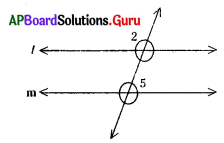A) parallel
B) Intersecting
C) perpendicular
D) can’t be determined
B) Intersecting

Question 30.
From the given angles are?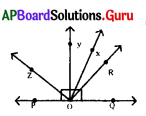A) ⌊ZOY & ⌊ROX
B) ⌊ZOP & ⌊ROQ
C) ⌊POY & ⌊QOY
D) ⌊ROX & ⌊XOY
C) ⌊POY & ⌊QOYQuestion 31.
Which of the following is not a vertically opposite angle?
A) Angles between legs of a folding cot
B) Angles between scissors
C) Angles between door and wall
D) Angles between legs of folding a chair
C) Angles between door and wall

Question 32.
The angles which have common arm are called ……………… angles.
A) interior
B) exterior
C) vertically opposite angles

Question 33.
The sum of two angles is 90°. Then they are called …………… angles.
A) Reflex
B) Straight
C) Complementary
D) Supplementary
C) Complementary

Question 34.
The sum of two angles is 180°. Then they are called …………… angles.
A) Supplementary
B) Complementary
C) Reflex
D) Complete
A) Supplementary

Question 35.
If two or more lines meet in a single point then the lines are called concurrent lines. The point where they intersect is called
A) Intersecting point
B) Point of contact
C) Orthocentre
D) Centroid
B) Point of contact

Question 36.
From the given figure $$\overline{\mathbf{O A}}$$, $$\overline{\mathbf{O B}}$$ are called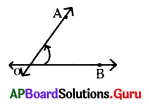A) angles
B) rays
C) arms
D) lines
C) arms

Question 37.
Supplementary of an angle is three times the angle then the angle is
A) 60°
B) 45°
C) 40°
D) 90°
A) 60°Question 38.
From the given figure ⌊a : ⌊b = 2 : 1 then ⌊c – ⌊d = ?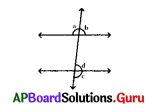A) 0°
B) 90°
C) 120°
D) 60°
D) 60°

Question 39.
From the given figure ⌊p : ⌊q = 2 : 3 then ⌊r = ?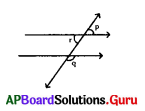A) 144°
B) 36°
C) 72°
D) 108°
C) 72°

Question 40.
From the given figure ⌊x = ?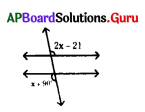A) 111°
B) 101°
C) 112°
D) 79°
A) 111°

Question 41.
From the given figure ⌊x = ?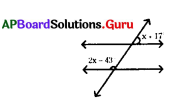A) 60°
B) 50°
C) 70°
D) 90°
A) 60°

Question 42.
AB line segment is denoted by
A) $$\stackrel{\leftrightarrow}{\mathrm{AB}}$$
B) • AB
C) $$\overline{\mathrm{AB}}$$
D) $$\overrightarrow{\mathrm{AB}}$$
C) $$\overline{\mathrm{AB}}$$

Question 43.
AB ray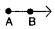is denoted by
A) $$\overleftarrow{\mathrm{AB}}$$
B) $$\overrightarrow{\mathrm{AB}}$$
C) $$\overleftrightarrow{\mathrm{AB}}$$
D) $$\overline{\mathrm{AB}}$$
B) $$\overrightarrow{\mathrm{AB}}$$

Question 44.
Which of the following are not pairs of complementary angles?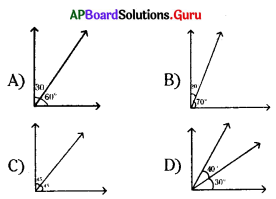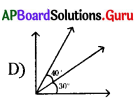Question 45.
Which of the following are not adjacent angles?